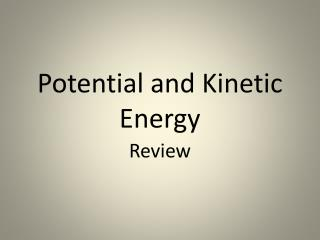Download PresentationPotential and Kinetic Energy

# Potential and Kinetic Energy

Download Presentation## Potential and Kinetic Energy

- - - - - - - - - - - - - - - - - - - - - - - - - - - E N D - - - - - - - - - - - - - - - - - - - - - - - - - - -
##### Presentation Transcript

1. Classify the following as a type of potential energy or kinetic energy (use the letters K or P) • _____ 1. A bicyclist pedaling up a hill _____ 2. An archer with his bow drawn _____ 3. A volleyball player spiking a ball _____ 4. A baseball thrown to second base _____ 5. The chemical bonds in sugar _____ 6. The wind blowing through your hair _____ 7. Walking down the street _____ 8. Sitting in the top of a tree _____ 9. A bowling ball rolling down the alley _____ 10. A bowling ball sitting on the rack

2. 11. Determine the kinetic energy of a 1000-kg roller coaster car that is moving with a speed of 20.0 m/s.

3. 12. A cinder block is sitting on a platform 20 m high. It weighs 79 N. The block has _____________ energy. Calculate it

4. 13. A 75-kg refrigerator is located on the 70th floor of a skyscraper (300 meters above the ground) What is the potential energy of the refrigerator?

5. 14. There is a bell that is 75 meters off the ground and can produce 12,000J when it falls. What is the mass of the bell?

6. 15. The potential energy of a 40-kg cannon ball is 14000 J. How high was the cannon ball to have this much potential energy?

7. 16. You serve a volleyball with a mass of 2.1 kg. The ball leaves your hand with a speed of 30 m/s. The ball has how much kinetic energy does it have?

8. 17. A car is traveling with a velocity of 40 m/s and has a mass of 1120kg. Find the amount of kinetic energy.

9. 18. What is the kinetic energy of a 3-kilogram ball that is rolling at 2 meters per second?

10. 19. An object has a K.E. of 45 J and a velocity of 3 m/s. What is the mass?

11. 20. An object with a mass of 2 kg has a K.E. of 36 J. What is the velocity?